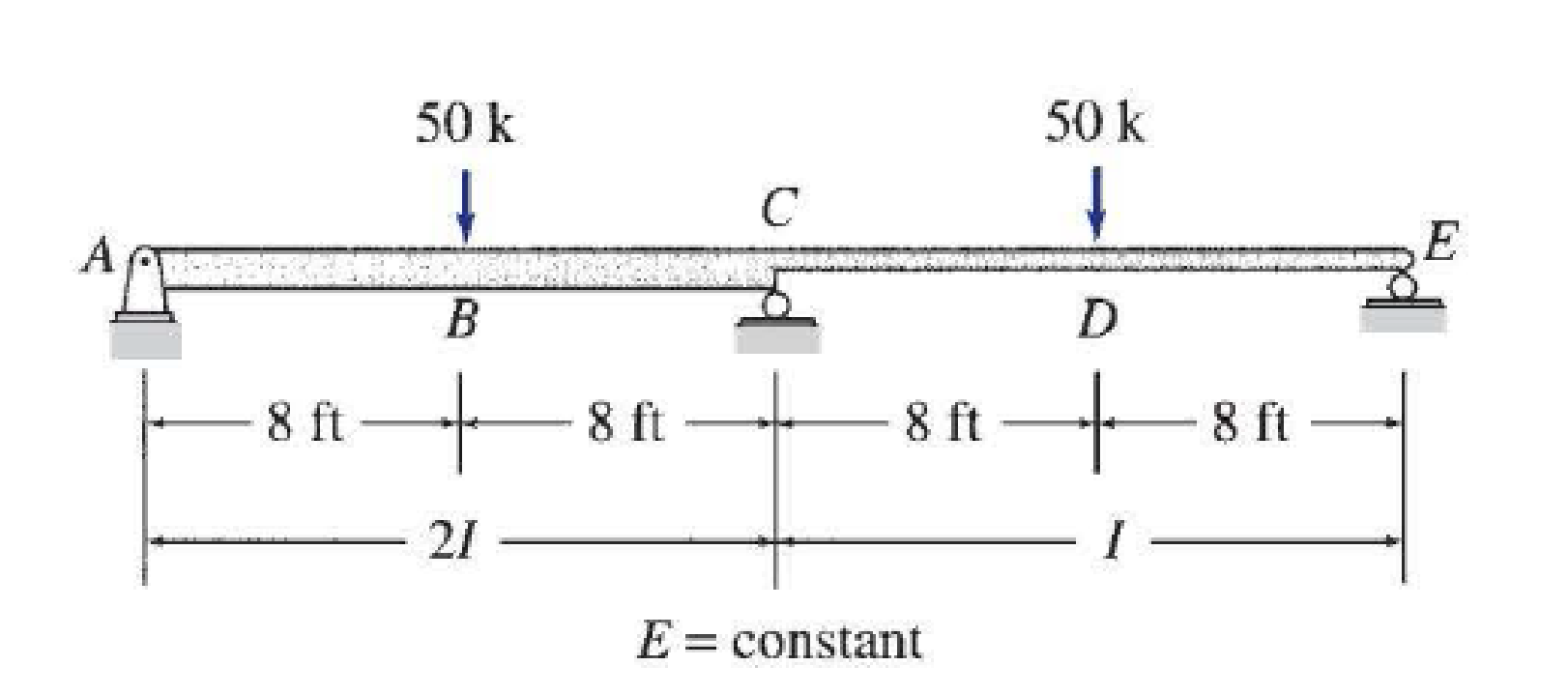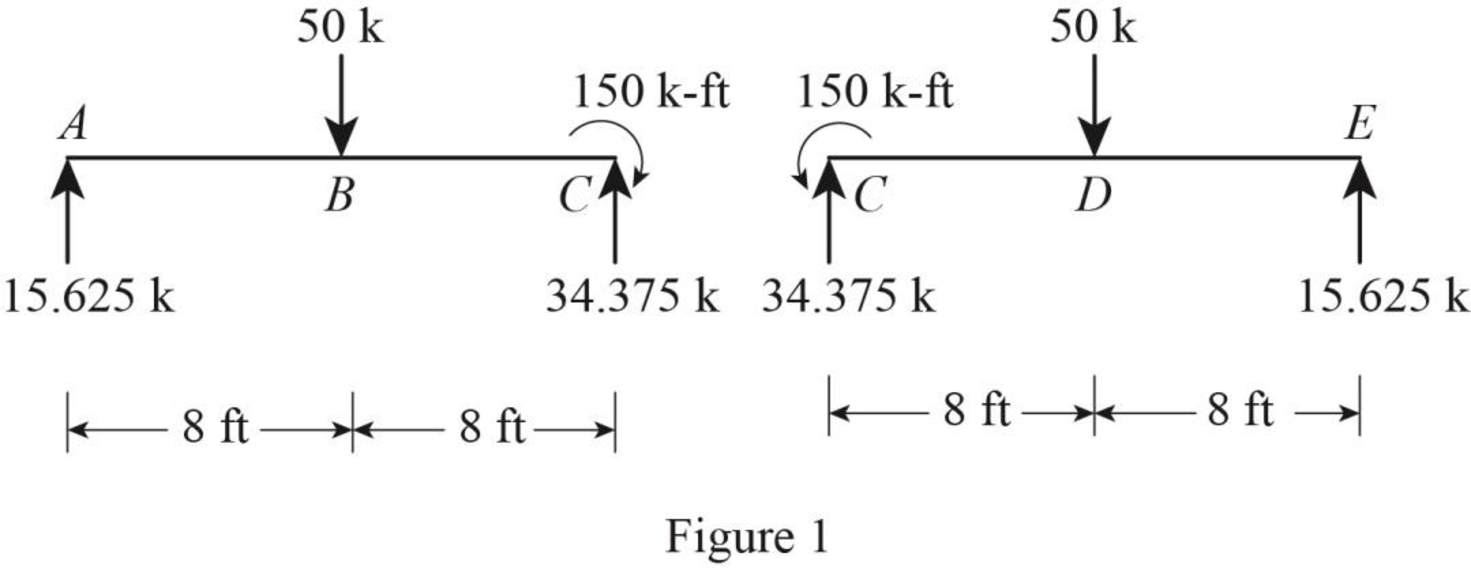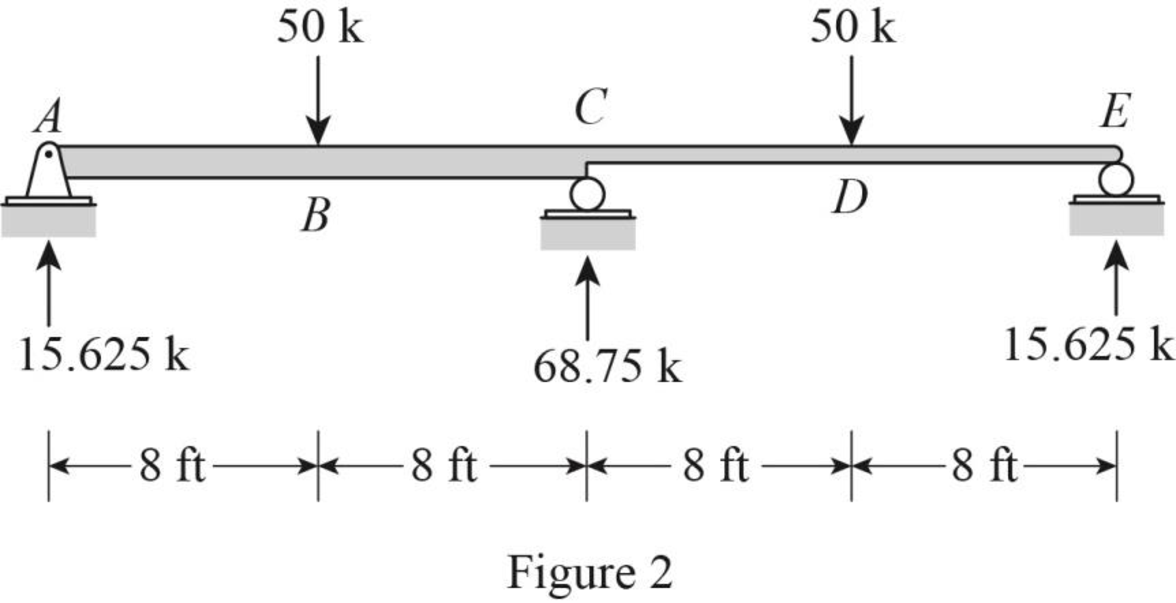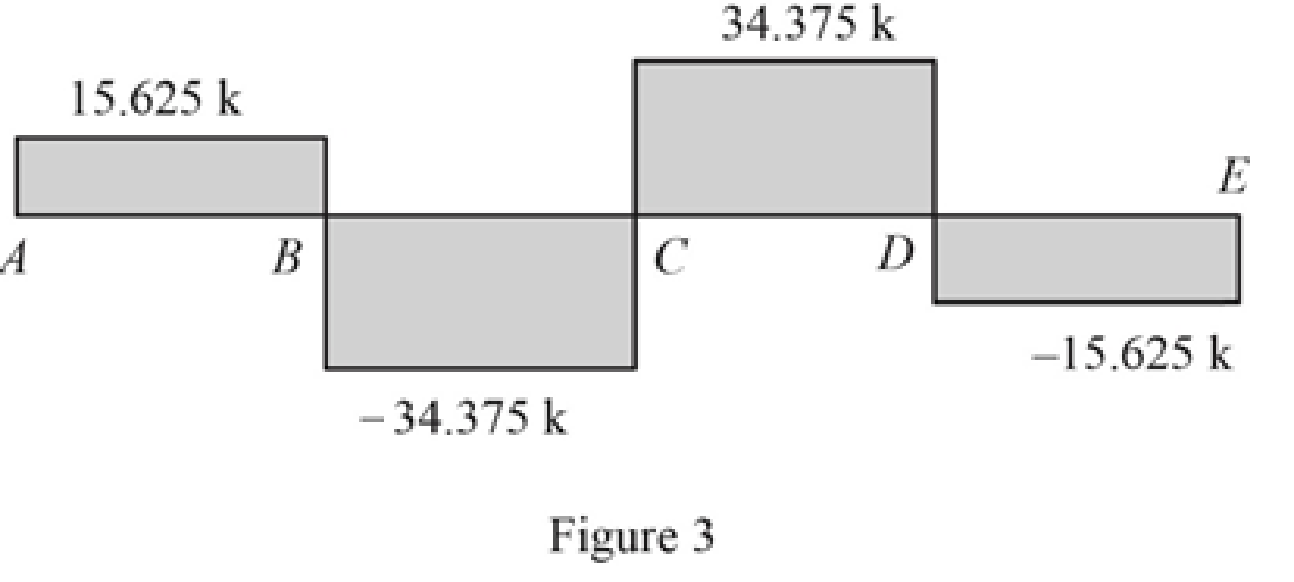# Determine the reactions and draw the shear and bending moment diagrams for the beams shown in Figs. PD.1 through PD.8 using the three-moment equation.BuyFind

### Structural Analysis

6th Edition
KASSIMALI + 1 other
Publisher: Cengage,
ISBN: 9781337630931BuyFind

### Structural Analysis

6th Edition
KASSIMALI + 1 other
Publisher: Cengage,
ISBN: 9781337630931

#### Solutions

Chapter D, Problem 1P
Textbook Problem

## Determine the reactions and draw the shear and bending moment diagrams for the beams shown in Figs. PD.1 through PD.8 using the three-moment equation.Expert Solution
To determine

Calculate the reactions for the given beam.

Sketch the shear and bending moment diagrams for the given beam.

## Answer to Problem 1P

The horizontal reaction at A is Ax=0_.

The vertical reaction at A is Ay=15.63k_.

The vertical reaction at C is Cy=68.75k_.

The vertical reaction at E is Ey=15.63k_.

### Explanation of Solution

Given information:

The structure is given in the Figure.

Apply the sign conventions for calculating reactions using the three equations of equilibrium as shown below.

• For summation of forces along x-direction is equal to zero (Fx=0), consider the forces acting towards right side as positive (+) and the forces acting towards left side as negative ().
• For summation of forces along y-direction is equal to zero (Fy=0), consider the upward force as positive (+) and the downward force as negative ().
• For summation of moment about a point is equal to zero (Matapoint=0), consider the clockwise moment as negative and the counter clockwise moment as positive.

Calculation:

Find the degree of indeterminacy of the structure:

Degree of determinacy of the beam is equal to the number of unknown reactions minus the number of equilibrium equations.

The beam is supported by 4 support reactions and the number of equilibrium equations is 3.

Therefore, the degree of indeterminacy of the beam is i=1.

Select the bending moment MB at the interior support B as redundant.

Consider the supports A, C, and E as l, c, and r respectively.

Express the general three-moment equation as shown below:

MlLlIl+2Mc(LlIl+LrIr)+MrLrIr=[PlLl2klIl(1kl2)PrLr2krIr(1kr2)wlLl34IlwrLr34Ir6E(ΔlΔcLl+ΔrΔcLr)]        (1)

Here, Mc is the bending moment at support c, Ml,Mr are the bending moments at the adjacent supports to the left and to the right of c, E is the modulus of elasticity, Ll,Lr are the lengths of the spans to the left and to the right of c, Il,Ir are the moments of inertia of the spans to the left and to the right of c, Pl,Pr are the concentrated loads acting on the left and the right spans, kl or kr is the ratio of distance of Pl or Pr from the left or right support to the span length, wl,wr are the uniformly distributed loads to the left and right spans, Δc is the settlement of support c, Δl,Δr is the settlement of adjacent supports to the left and to the right of c,

Refer the given Figure.

The moment at A and E is MA=ME=0.

Apply three-moment equation at joint C,

Substitute 16 ft for Ll, 16 ft for Lr 2I for Il, I for Ir, 50 k for Pl, 50 k for Pr, 0.5 for kl, 0.5 for kr, 0 for wl, 0 for wr, and 0 for Δc,Δl,Δr in Equation (1).

2MC(162I+16I)=50(16)2(0.5)2I(10.52)50(16)2(0.5)I(10.52)48MCI=2,400I4,800I48MC=7,200MC=150k-ft

Sketch the span end moments and shears for span ABC and CDE as shown in Figure 1.Use equilibrium equations:

For span ABC,

Summation of moments of all forces about A is equal to 0.

MA=0Cy(16)15050(8)=0Cy=34.375k

Summation of forces along y-direction is equal to 0.

+Fy=0Ay50+Cy=0Ay50+34.375=0Ay=15.625k

Ay15.63k

For span CDE,

Summation of moments of all forces about C is equal to 0.

MC=0Ey(16)+15050(8)=0Ey=15.625kEy15.63k

Summation of forces along y-direction is equal to 0.

+Fy=0Cy50+Ey=0Cy50+15.625=0Cy=34.375k

Find the total reaction at C.

Cy=34.375k+34.375k=68.75k

Sketch the reactions for the given beam as shown in Figure 2.Find the shear force (S) for the given beam:

At point A,

SA,R=15.625k

At point B,

SB,L=15.625kSB,R=15.62550=34.375k

At point C,

SC,L=15.62550=34.375kSC,R=15.62550+68.75=34.375k

At point D,

SD,L=15.62550+68.75=34.375kSD,R=15.62550+68.7550=15.625k

At point E,

SE,L=15.62550+68.7550=15.625k

Sketch the shear diagram for the given beam as shown in Figure 3.Find the bending moment (M) for the given beam:

At point A,

MA=0

At point B,

MB=15.625(8)=125k-ft

At point C,

MC=15.625(16)50(8)=150k-ft

At point D,

MD=15.625(24)50(16)+68.75(8)=125k-ft

At point E,

ME=0

Sketch the bending moment diagram for the given beam as shown in Figure 4.### Want to see more full solutions like this?

Subscribe now to access step-by-step solutions to millions of textbook problems written by subject matter experts!

### Want to see more full solutions like this?

Subscribe now to access step-by-step solutions to millions of textbook problems written by subject matter experts!

#### Additional Engineering Textbook Solutions

Find more solutions based on key concepts
Which layer of the Earth contains the most amount of mass?

Engineering Fundamentals: An Introduction to Engineering (MindTap Course List)

What is a subtype discriminator? Give an example of its use.

Database Systems: Design, Implementation, & Management

Identify the six basic components of any computer-based information system.

Principles of Information Systems (MindTap Course List)

What are some capabilities of artificial intelligence?

Enhanced Discovering Computers 2017 (Shelly Cashman Series) (MindTap Course List)

What must be known to use the compound-rest taper turning method?

Precision Machining Technology (MindTap Course List)

Voltage can be described as ____________ .

Welding: Principles and Applications (MindTap Course List)

If your motherboard supports ECC DDR3 memory, can you substitute non-ECC DDR3 memory?

A+ Guide to Hardware (Standalone Book) (MindTap Course List)© 2021 bartleby. All Rights Reserved.